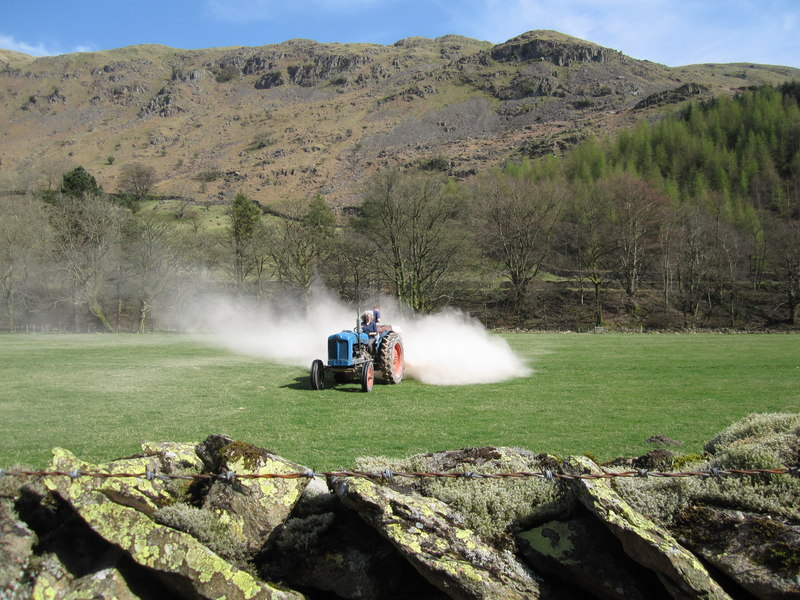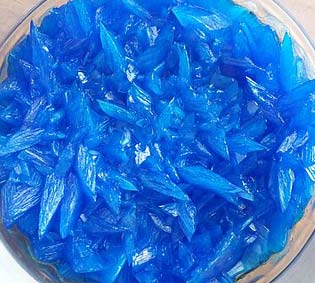Chemistry CIE iGCSE:
Acids, Bases + Salts 1

Page 1

1. Rain water is weakly acidic because it contains dissolved carbon dioxide. Which of these is a possible pH for rain water?

2. The products of the reaction between magnesium and hydrochloric acid are ...
• A.   magnesium chloride and water
• B.   magnesium chloride and hydrogen
• C.   magnesium chlorate and water
• D.   magnesium chlorate and hydrogen
3. Four solutions with different pH values were tested with universal indicator.

Which row shows the colour of each solution after the indicator was added?

 pH 9 pH 1 pH7 pH6 A purple red yellow orange B red yellow green blue C blue red green yellow D blue green yellow orange
4. Slaked lime (calcium hydroxide) is used to neutralize acidic soils.Which ion is responsible for neutralizing the acid in the soil?
• A.   Ca2+
• B.   H+
• C.   OH-
• D.   O2-
5. Which is NOT a typical characteristic of acids?
• A.   They react with metal oxides producing hydrogen
• B.   They react with carbonates producing carbon dioxide
• C.   They react with alkalis producing water
• D.   They turn blue litmus paper red

6. The pH values of four aqueous solutions are:

 Solution pH P 13 Q 7 R 2 S 9

Which of these solutions are alkaline?

• A.   R only
• B.   R and Q only
• C.   Q, S and P only
• D.   S and P only

Q7-10:
A student prepared a sample of hydrated copper(II)sulfate crystals by reacting excess copper(II)carbonate with dilute sulfuric acid.7. The equation for this reaction with the correct state symbols is ..
• A.  CuCO3(s) + H2SO4(l)  CuSO4(l) + H2O(l) + CO2(g)
• B.  CuCO3(s) + H2SO4(aq)  CuSO4(aq) + H2O(l) + CO2(g)
• C.  CuCO3(s) + H2SO4(l)  CuSO4(aq) + H2O(l) + CO2(g)
• D.  CuCO3(aq) + H2SO4(aq)  CuSO4(aq) + H2O(l) + CO2(g)
8. How would the student know when she had added an excess of copper(II)carbonate?
• A.   the solution would turn blue in colour
• B.   the temperature would rise
• C.   effervescence would stop and solid would remain in the mixture
• D.   all the solid would disappear

9. What techniques would the student use to:

(a) separate the excess copper(II)carbonate from the mixture?
(b) obtain hydrated copper(II)sulfate crystals from the solution?

 Separate excess copper(II) carbonate Obtain hydrated copper(II)sulfate crystals A filtration crystallisation B evaporation distillation C filtration distillation D evaporation crystallisation
10. Why was excess copper(II)carbonate used in the salt preparation?
• A.   To ensure that a reaction takes place
• B.   To speed up the reaction
• C.   To allow the solid to be separated from the solution
• D.   To ensure that all the acid is used up